# Kinematics of robot manipulators - inverse kinematics

In the previous two tutorials we examined methods of forward kinematics for a six axis robot model where we computed the real world co-ordinates of the tool tip for known joint angles.  In this tutorial we consider the problem in the opposite direction, that is we know the intended co-ordinates of the end effector and from this information compute the joint angles.  This procedure is called inverse kinematics.

Unlike forward kinematics, there is no generic solution for inverse kinematics that can be applied to all configurations of robot manipulators.  Text books generally state three categories of solutions:

• geometric
• analytic
• numerical

Numerical solutions apply iterative processes requiring complex software and are outside the scope of this series of tutorials.

Geometric methods are straightforward where there are a limited number of links. We present an example using the robot model to give a complete solution but only for a limited range of orientations of the tool tip.

Analytic solutions exist in varying degrees of complexity, depending on the number of joints but there is no single generic solution for a six axis robot.  We will outline a method using transformation matrices that is valid under certain conditions.

### Geometric method

Fig.1 below shows a six axis robot model similar to the one used in the tutorials for forward kinematics. However, in this model there are no offsets between joints 0 & 1,  joints 1 & 2 and joints 2 & 3.  Also, the rotational axes of joints 3, 4 and 5 now intersect to provide a spherical wrist configuration for the final three joints.  Note that the origins of co-ordinate frames 3, 4 and 5 are now coincident; which assists the analytical method for inverse kinematics explained later.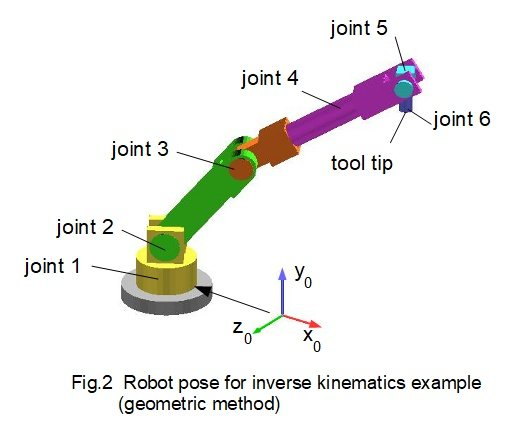The basis for this example is the pose for the tool tip shown above in Fig.2.  The co-ordinates of the origin of frame 5* relative to the base frame are (in unspecified length units): x5 = 695, y5 = 781, z5 = -401.  The tool tip is 110 units vertically below this point.  In this configuration joint 4 and joint 6 do not rotate.  Consequently joint angle θ5 is determined by joint angles θ2 and θ3.  In effect, this configuration has three degrees of freedom.

* These co-ordinates are also the origin of frames 4 and 6.  The choice of frame 5 to designate this point is arbitrary.

Fig.3 below illustrates the geometry of the robot in the required configuration with the joints aligned with the X axis in the datum position. Note that the "elbow" at joint 3 is in the up position. The inverse kinematic problem is to find joint angles θ1, θ2, θ3 5For  θ1

From the top view θ1 = tan-1(z5 / x5) = tan-1(401/ 695)        gives     θ1 = 29.98°    The diagram confirms rotation is +ve (Y0 axis coming out the page)

For  θ2

From the top view    r = z5 / sin θ1  = (401) / sin (29.98°) = 802

From the side view on direction A    tan-1φ = tan-1(y5 - a1) / r) = tan-1(621 / 802)    gives φ = 37.74°

Also       L12 = r2 + (y5 - a1)2 = (802)2 + (781 - 160)2

gives    L1 = √[(802)2 + (621)2 ] = 1015L2

From the robot model    L2 = 400    L3 = 630

Using the law of cosines for triangle with sides L1, L2, L3 and internal angles λ1 λ2 λ3   gives     L32 = L12 + L22 - 2L1.L2.cos λ3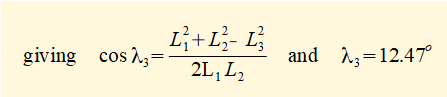and thus     θ2  =  (φ + λ3 ) =  (37.74° + 12.47°) = 50.21°     The diagram confirms rotation is +ve (Z1 axis coming out the page)

For  θ3

Using the law of cosines for triangle with sides L1, L2, L3 and internal angles λ1 λ2 λ3   gives   L12 = L22 + L32 - 2L2.L3.cos λ1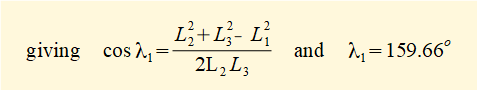gives  θ3 = (180° -  λ1 ) = (180° - 159.66°) = 20.34°     The diagram shows joint rotation is -ve. Thus  θ3 = - 20.34°

For  θ5

β = (θ2 - θ3) = (50.21° - 20.34°) = 29.87°   and     θ5 -= (90° + β)    gives    θ5  = 119.87°

The diagram shows joint rotation is -ve. Thus  θ5  = - 119.87°

However, this solution for the joint angles is not unique.  Fig.4 below illustrates another valid solution where the elbow joint is pointing down.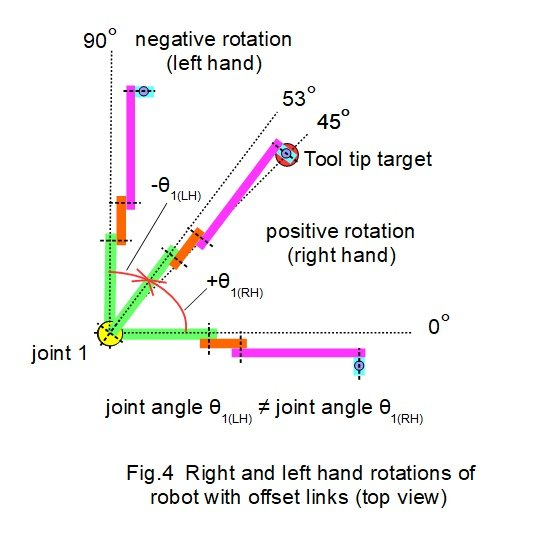In the elbow down configuration:

θ2  =  (φ - λ3 ) = 25.27° (+ve rotation)

θ3  = 20.34° (+ve rotation)

θ5 = (β  +  θ3   +   90°) = (θ2  +  θ3   +   90°)  = 135.61° (-ve rotation)

Other variable aspects of the robot configuration, such as offsets, introduce multiple inverse kinematic solutions.  The robot model in the example above has no offsets but consider Fig.5 below where links are offset.When the arms rotate towards the target from opposite sides the joint angles are not the same.  As a consequence inverse kinematics must define the direction of rotation, normally stated as right hand or left hand.

We carry forward the above solution for joint angles θ1, θ2, θ3  to the next section as part of an inverse kinematic solution where all six joint angles rotate.

### Analytic method

#### Procedure

The analytic method described below treats the inverse kinematics solution for a six axis robot in two parts.

Firstly, a solution for joint angles θ1, θ2, θ3  is found.  For this example we used the geometric method outlined above to find θ1, θ2, θ3  We then derive rotation matrix R03 and insert the values of θ1, θ2, θ3

The second part requires joints 4, 5 and 6 to be in a spherical wrist configuration (see above).  Because of this, rotation matrix R36 alone defines transformations  between co-ordinate frame 3 and co-ordinate frame 6.  The elements of R36 are expressed in terms of sine and cosine functions of joint angles θ4, θ5, θ6

We specify the orientation of co-ordinate frame 6 which defines the orientation of the tool tip and also defines rotation matrix R06 which is the rotational transformation between the base frame and frame 6.

Now a clever bit of linear algebra:

R06 = R03 . R36

multiply both sides by R03-1 (the inverse of R03)    gives    R03-1.R06 = R03-1.R03 . R36

as  R03-1.R03  = I (identity matrix) it follows    R36  =   R03-1.R06

We then compute R03-1.R06   and  use the equivalence with previously determined matrix R36  to solve for θ4, θ5, θ6

#### Example

We will work through this procedure using the parameters of the previous example (elbow up). For convenience joint angles are rounded to: θ1 = 30° (+ve), θ2 = 50° (+ve), θ3 = 20° (-ve).

Rotation matrix R03

R01 is rotation of +30° about the Y axis;    R12 is rotation of +50° about the Z axis;     R23 is rotation of -20° about the Z axis;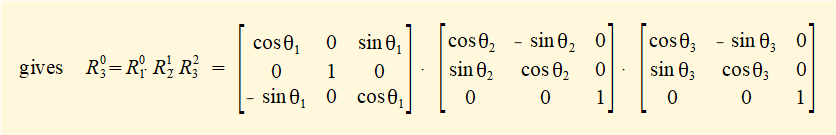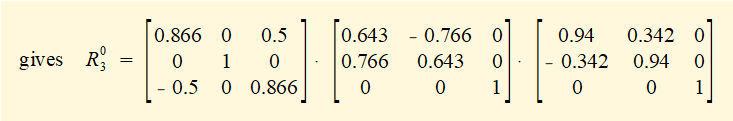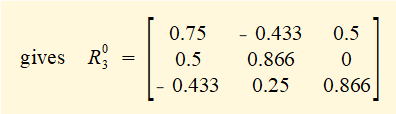The inverse of a rotation matrix is its transpose, thus: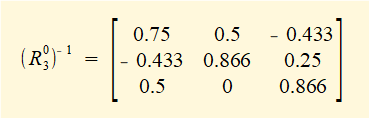Rotation matrix R06

Fig.6 below shows the orientation of the tool tip at datum position (frame 0) and at the required pose (frame 6).  Column vectors at frame 6 show transformations to frame 0 by application of rotation matrix R06 .  We deduce these column vectors directly from the respective co-ordinate axes.  In this case the X0 and Z0 axes are rotated through 90°.  Finding column vectors for rotations through other angles would require  a more detailed diagram.This stage provides good insight into transformations between reference frames.  R06  transforms vectors in frame 6 to vectors in frame 0.Compute rotation matrix R36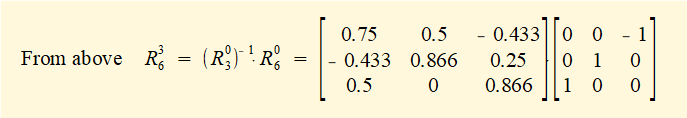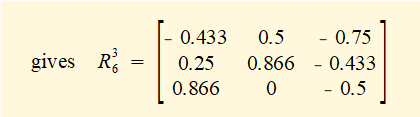Derive rotation matrix R36  from first principles

R34 is rotation of θ4 about the X4 axis;    R45 is rotation of θ5 about the Z5 axis;     R56 is rotation of θ6 about the X6 axis;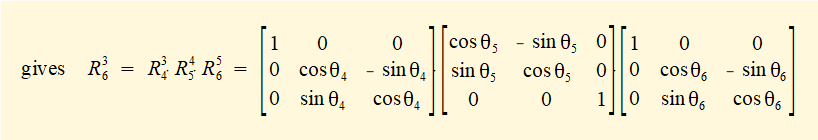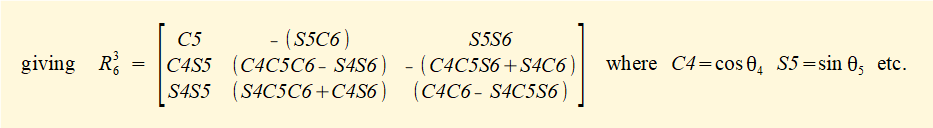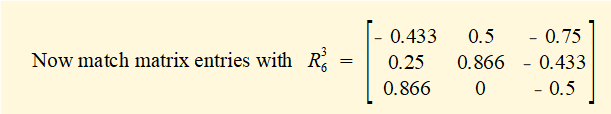This next stage is not entirely straightforward since multiple solutions are possible for each element. First consider the element r11

cosθ5 = - 0.433    There are two possible solutions:  θ5 =  (i) cos-1(-0.433) = 115.7°    (ii) cos-1(-0.433) = -115.7°

Choose θ5  = -115.7°  giving  sinθ5  = - 0.901

Consider element r12    - (sinθ5 cosθ6) = 0.5

gives cosθ6  =   - 0.5 / (- 0.901) = 0.555   thus  θ6  =  cos-1(0.555) =  (i) 56.3°  or (ii) - 56.3°

Choose  θ6  = 56.3°    giving  sinθ6  = 0.832

Check element r13        sinθ5 sinθ6  = (- 0.901) (0.832) = - 0.75 (correct)      choosing  θ6  = - 56.3° would be incorrect as sin( - 56.3°) is -ve

Consider element r21 = cosθ4 sinθ5

gives  cosθ4  = 0.25 / ( - 0.901) = - 0.277      thus θ4 = cos-1(- 0.277) = (i) 106.1° or  (ii) - 106.1°

Choose  θ4  = - 106.1°    giving  sinθ4  = - 0.961

Check element r31         sinθ4 sinθ5  = (- 0.961) (- 0.901) = 0.866 (correct)      choosing  θ4  = 106.1° would be incorrect as sin(106.1°) is +ve

To conclude:     θ4  = - 106.1°         θ5  = -115.7°      θ6  = 56.3°

Elements r22 r23 r32 r33 provide check sums to validate this solution.

A valid solution also occurs for  θ5  = + 115.7° the resulting joint angles being  θ4  = - 73.9°         θ5  = 115.7°      θ6  = -123.7°

Fig.7 below shows the robot model: (a) at datum position, (b) pose with joint angles from this example, (c) top view of pose showing orientation of the tool tip as defined in Fig.6.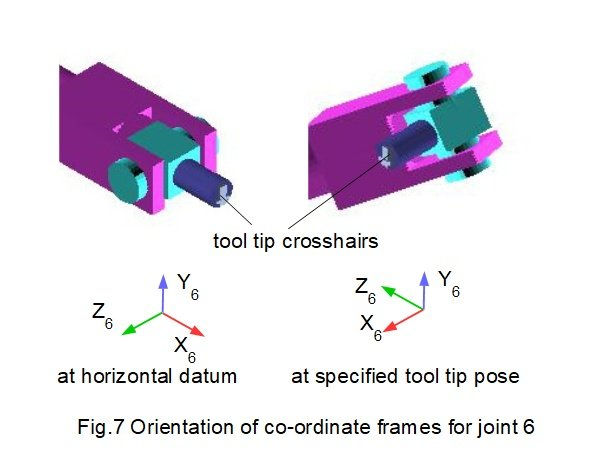I welcome feedback at:

### Tutorials - mechanical vibrations

###### Forced vibrations with damping

Alistair's tutorials 2021